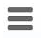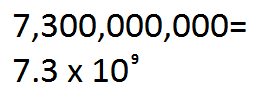﻿ Scientific Notation Converter ﻿# Scientific Notation Converter

### Convert Any Number to Scientific NotationThis Scientific Notation Converter converts the number which the user enters into scientific notation form.

The scientific notation of a number is a number between 1.0 and 9.99999 multiplied by the base of 10 raised to an exponent.

Scientific notation is very useful for making very large or very small numbers more easily readable and, thus, understandable.

As an example, let's take the number 0.0000000000000000000056. This is not easily read. This same number in scientific notation is 5.6 x 10-21. Now it is much easier for scientists to read this number. It's is easier quantified terms now. Let's take now a very large number, such as 23,000,000,000,000,000. This number in scientific notation is 2.3 x 1016. This, again, is much easier to read and understand.

This is why scientific notation is so important and why it's used and why you should know how to convert or have a convert available to make numbers be in scientific notation form.

In order to be in scientific form, the number which will be multiplied to a base 10 number and exponent must be betweeen 1.0 and 9.999. This is the first rule of scientific notation. Once the number is between 1 and 10, we then multiply by base 10. The exponent of the base 10 number determines how large or small the number is. If the exponent is negative, then the end value will be a number smaller than 1. If the exponent is 0, the number retains its original value. If the exponent is positive, then the number will be larger than 10.

To use this converter/calculator, a user just enters the number which he or she wants to scientific notation and clicks the 'Calculate' button. The scientific notation of the number will then automatically be computed and shown.

Again, scientific notation is a form heavily used in the scientific community to making numbers more easily understandable.

Example Calculations

Compute the scientific notation of the number 0.0000023